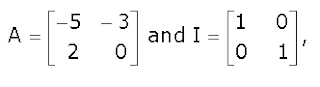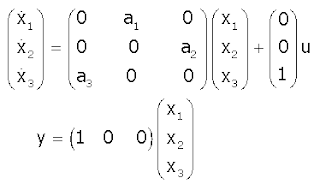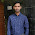## Control System Solved Competitive Questions:

 An open loop system represented by the transfer function G(s) = (s-1) / (s+2)(s+3) is
(a) Stable and of the minimum phase type
(b) Stable and of the non-minimum phase type
(c) Unstable and of the minimum phase type
(d) Unstable and of the non-minimum phase type

 The open loop transfer function G(s) of a unity feedback control system is given as,
G(s) = [ k(s+2/3)  /  s2(s+2) ]
From the root locus, it can be inferred that when k tends to positive infinity,
(a) Three roots with nearly equal real parts exist on the left half of the s-plane
(b) One real root is found on the right half of the s-plane
(c) The root loci cross the jω axis for a finite value of k; k≠0
(d) Three real roots are found on the right half of the s-plane

 Given thatthen the value of A3 is  [GATE2012]
(a) 15A+12I
(b) 19A+30I
(c) 17A+15I
(d) 17A+21I

 The matrix [A]=is decomposed into a product of a lower triangular matrix [L] and an upper triangular matrix [U]. The properly decomposed[L] and [U] matrices respectively are............The options A,B,C,D are given below.

 The input x(t) of a system are related as y(t) =  ∫t-∞ x(τ)cos(3τ)dτ. The system is [GATE2012]
(a) Time-invariant and stable
(b) Stable and not time-invariant
(c) Time-invariant  and not stable
(d) Not time-invariant and not stable

The feedback system shown below oscillates at 2 rad/s when [GATE2012](a) k=2 and a=0.75
(b) k=3 and a=0.75
(c) k=4 and a=0.5
(d) k=2 and a=0.5

 The Fourier transform of a signal h(t) is H(jω) = (2cosω)(sin2ω)/ω. The value of h(0) is [GATE2012]
(a) 1/4
(b) 1/2
(c) 1
(d) 2

 The state variable description of an LTI system is given bywhere y is the output and u is the input.The system is controllable for, [GATE2012]
(a) a1≠0,a2=0,a3≠0
(b) a1=0,a2=0,a30
(c) a1=0,a2=0,a3=0
(d) a10,a20,a3=0

 The state transition diagram for the logic circuit shown is  [GATE2012]Given thatthen the value of A3 is  [GATE2012]
(a) 15A+12I
(b) 19A+30I
(c) 17A+15I
(d) 17A+21I

 The differentiator has a transfer function whose [Gate 1997]
(a) Phase increases linearly with frequency
(b) Amplitude remains constant
(c) Amplitude increases linearly with frequency
(d) Amplitude decreases linearly with frequency

 Introduction of integral action in the forward path of a unity feedback system results in a [Gate 1997]

(a) Marginally stable system
(b) System with no steady state error
(c) System with increase stability margin
(d) System with better speed of response

7:53 PM

#### 2 comments:

1.question No.11 is wrong as frequency increases magnitude increases and the phase remains constant so correct option is C

2.Question No.12 is wrong as integral action introduces error decreases and accuracy increases and the stability is decreases so the correct option is B